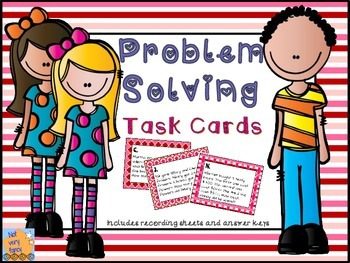# Valentine's Problem Solving Task CardsSubject
Resource Type
Format
PDF (1 MB|25 pages)
Standards
\$3.00
\$3.00

### Description

Give your students a real challenge with these Valentine's Day themed math problems!

There are 60 task cards with Valentine's themed problems written at 3 different levels. All different problem types are included. The three sets are identical except for the numbers and the frame. Each set has a corresponding recording sheet and answer key. For a bit of teacher humor, the subjects of all the problems are presidents and first ladies.

Don't miss out on this fabulous DOLLAR DEAL:
Valentine's Primary Math Packet
or these FREEBIES
: Valentine's Day Following Directions Lesson Valentine Themed Fact Sorting Math Center

. There are 138 task cards. Most tasks have several components, and many are open ended.

For more differentiated problem solving try this 20 problem set of Primary Problem Solving focusing on unknown addend word problems and compare problems.

St Patrick's Day and spring are not as far away as you think! Be ready with these great products:

Shamrock Place Value - free sample
St. Patrick's Day Themed Place Value
Spring Themed Place Value

Thanks for visiting my store!
Be sure to follow for information on freebies, sales, and new products.
I also blog at: Not very fancy

Deb
Total Pages
25 pages
N/A
Teaching Duration
N/A
Report this Resource to TpT
Reported resources will be reviewed by our team. Report this resource to let us know if this resource violates TpT’s content guidelines.

### Standards

to see state-specific standards (only available in the US).
Add and subtract within 20, demonstrating fluency for addition and subtraction within 10. Use strategies such as counting on; making ten (e.g., 8 + 6 = 8 + 2 + 4 = 10 + 4 = 14); decomposing a number leading to a ten (e.g., 13 - 4 = 13 - 3 - 1 = 10 - 1 = 9); using the relationship between addition and subtraction (e.g., knowing that 8 + 4 = 12, one knows 12 - 8 = 4); and creating equivalent but easier or known sums (e.g., adding 6 + 7 by creating the known equivalent 6 + 6 + 1 = 12 + 1 = 13).
Relate counting to addition and subtraction (e.g., by counting on 2 to add 2).
Solve word problems that call for addition of three whole numbers whose sum is less than or equal to 20, e.g., by using objects, drawings, and equations with a symbol for the unknown number to represent the problem.
Use addition and subtraction within 20 to solve word problems involving situations of adding to, taking from, putting together, taking apart, and comparing, with unknowns in all positions, e.g., by using objects, drawings, and equations with a symbol for the unknown number to represent the problem.